Equal - Multiplication

Chapter 4 Class 11 Mathematical Induction
Concept wise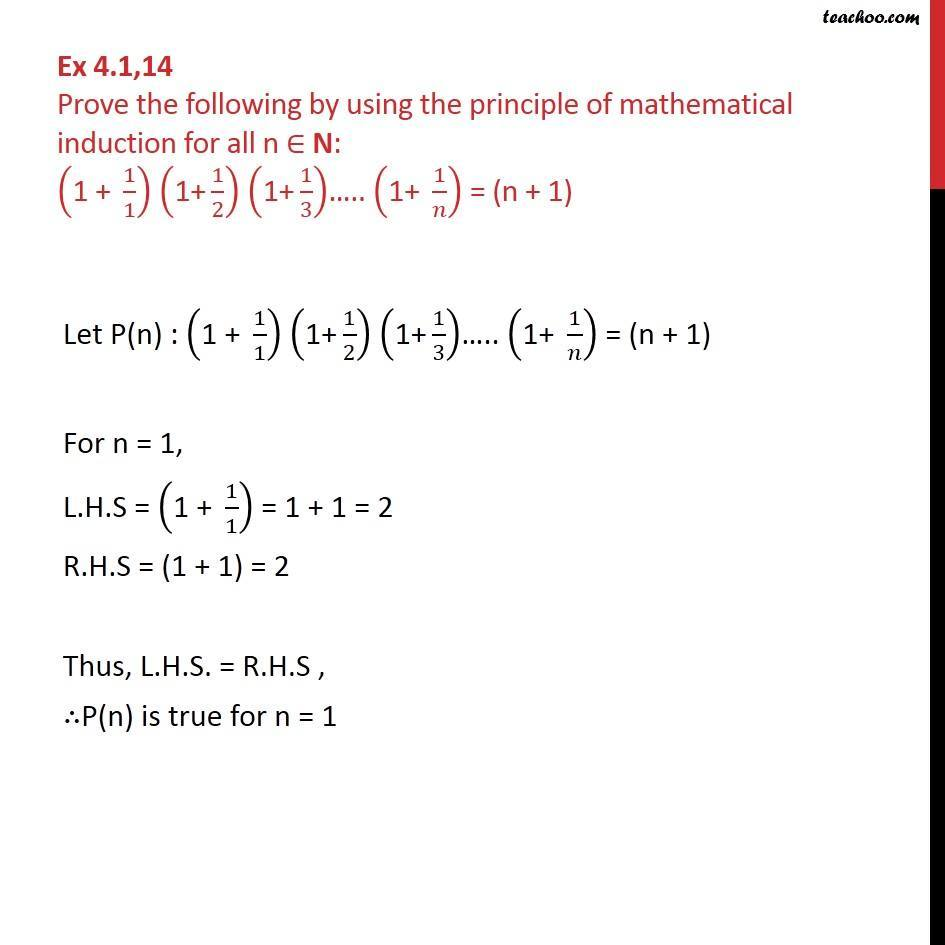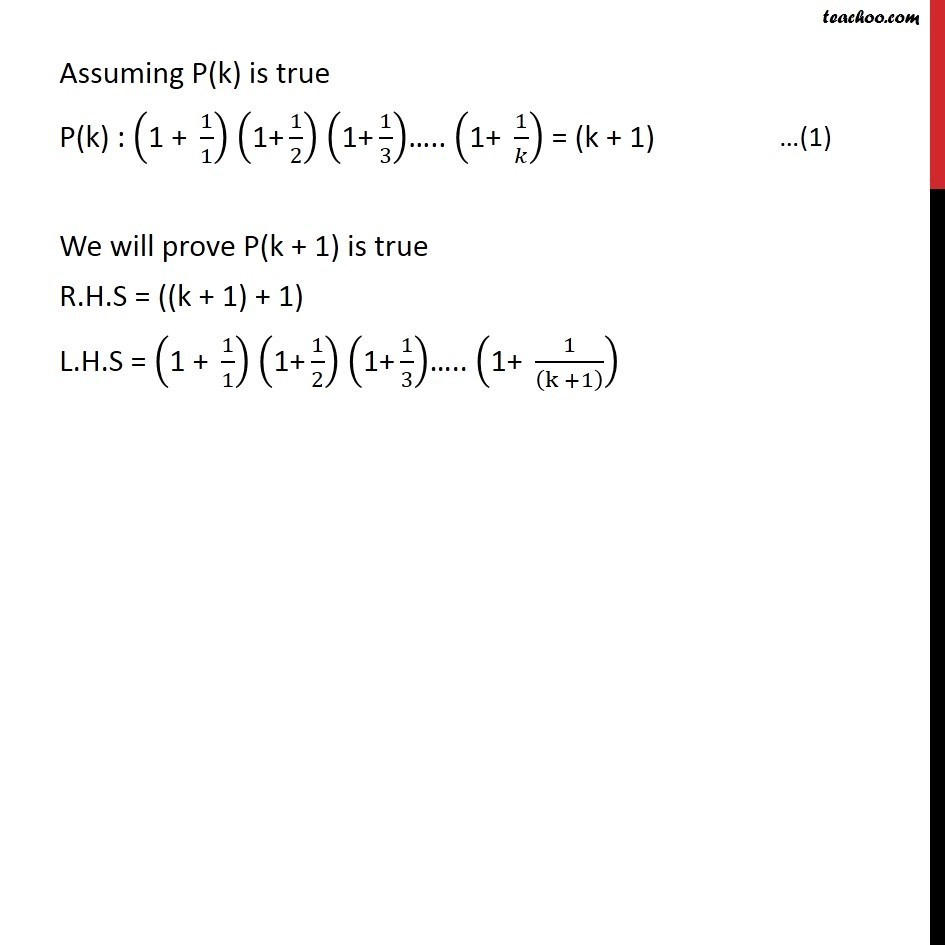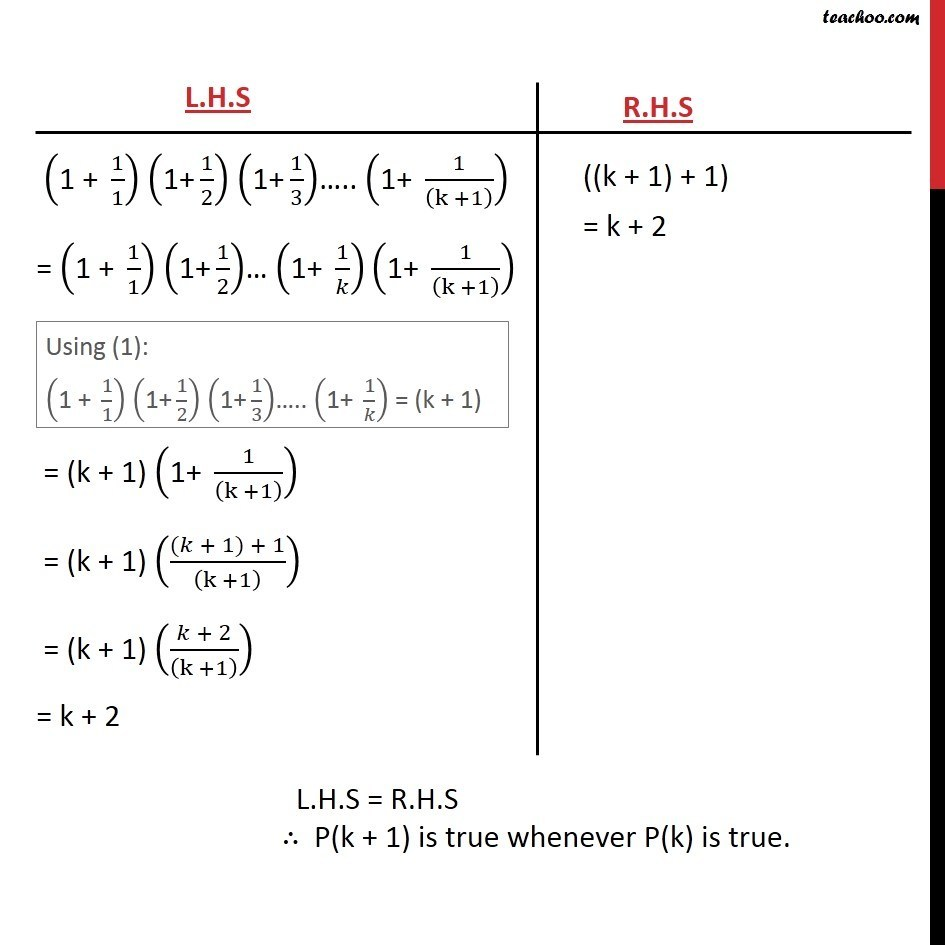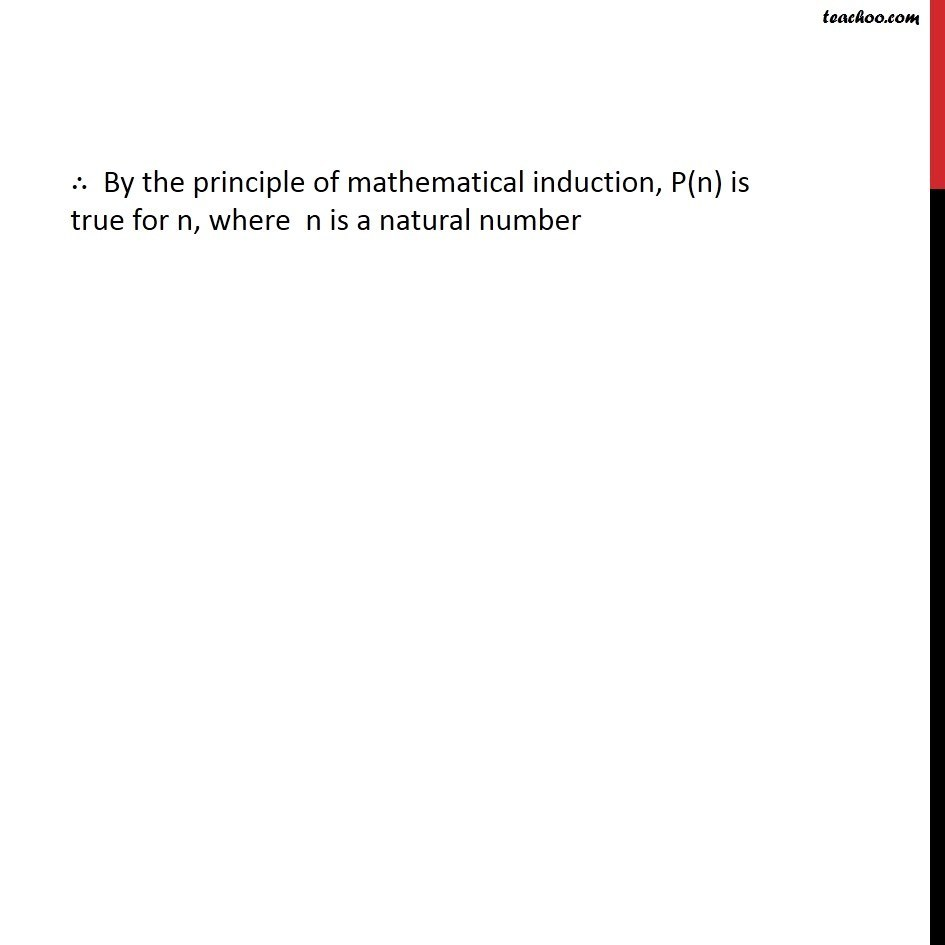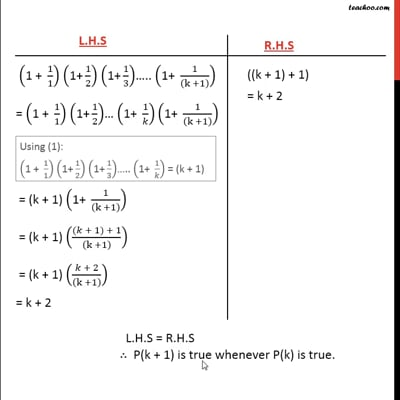This video is only available for Teachoo black users

Introducing your new favourite teacher - Teachoo Black, at only ₹83 per month

### Transcript

Ex 4.1,14 Prove the following by using the principle of mathematical induction for all n ∈ N: ("1 + " 1/1) ("1+" 1/2) ("1+" 1/3)….. ("1+ " 1/𝑛) = (n + 1) Let P(n) : ("1 + " 1/1) ("1+" 1/2) ("1+" 1/3)….. ("1+ " 1/𝑛) = (n + 1) For n = 1, L.H.S = ("1 + " 1/1) = 1 + 1 = 2 R.H.S = (1 + 1) = 2 Thus, L.H.S. = R.H.S , ∴P(n) is true for n = 1 Assuming P(k) is true P(k) : ("1 + " 1/1) ("1+" 1/2) ("1+" 1/3)….. ("1+ " 1/𝑘) = (k + 1) We will prove P(k + 1) is true R.H.S = ((k + 1) + 1) L.H.S = ("1 + " 1/1) ("1+" 1/2) ("1+" 1/3)….. ("1+ " 1/((k +1) )) ∴ By the principle of mathematical induction, P(n) is true for n, where n is a natural number